Groupoid

There are at least two definitions of groupoid'' currently in use.

The first type of groupoid is an algebraic structure on a Set with a Binary Operator. The only restriction on the operator is Closure (i.e., applying the Binary Operator to two elements of a given setreturns a value which is itself a member of). Associativity, commutativity, etc., are not required (Rosenfeld 1968, pp. 88-103). A groupoid can be empty. The numbers of nonisomorphic groupoids of this type havingelements are 1, 1, 10, 3330, 178981952, ... (Sloane's A001329), and the numbers of nonisomorphic and nonantiisomorphic groupoids are 1, 7, 1734, 89521056, ... (Sloane's A001424). An associative groupoid is called a Semigroup.

The second type of groupoid is an algebraic structure first defined by Brandt (1926) and also known as a Virtual Group. A groupoid with base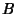is a setwith mappingsandfromontoand a partially defined binary operation, satisfying the following four conditions:

1.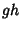is defined only whenfor certain mapsandfromontowithand2. Associativity: If eitheroris defined, then so is the other and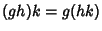.

3. For eachin, there are left and right Identity Elementsand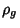such that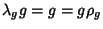.

4. Eachinhas an inverse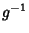for whichand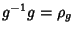(Weinstein 1996). A groupoid is a small Category with every morphism invertible.

See also Binary Operator, Inverse Semigroup, Lie Algebroid, Lie Groupoid, Monoid, Quasigroup, Semigroup, Topological Groupoid

References

Brandt, W. Über eine Verallgemeinerung des Gruppengriffes.'' Math. Ann. 96, 360-366, 1926.

Brown, R. From Groups to Groupoids: A Brief Survey.'' Bull. London Math. Soc. 19, 113-134, 1987.

Brown, R. Topology: A Geometric Account of General Topology, Homotopy Types, and the Fundamental Groupoid. New York: Halsted Press, 1988.

Higgins, P. J. Notes on Categories and Groupoids. London: Van Nostrand Reinhold, 1971.

Ramsay, A.; Chiaramonte, R.; and Woo, L. Groupoid Home Page.'' http://amath-www.colorado.edu:80/math/researchgroups/groupoids/groupoids.shtml.

Rosenfeld, A. An Introduction to Algebraic Structures. New York: Holden-Day, 1968.

Sloane, N. J. A. Sequences A001424 and A001329/M4760 in An On-Line Version of the Encyclopedia of Integer Sequences.'' http://www.research.att.com/~njas/sequences/eisonline.html and Sloane, N. J. A. and Plouffe, S. The Encyclopedia of Integer Sequences. San Diego: Academic Press, 1995.

Weinstein, A. Groupoids: Unifying Internal and External Symmetry.'' Not. Amer. Math. Soc. 43, 744-752, 1996.